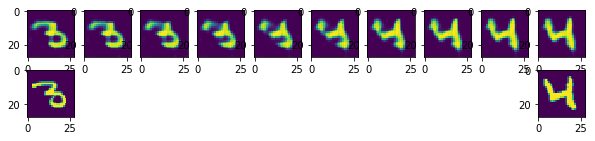# Transpose Convolutions and Autoencoders¶

In our discussions of convolutional networks, we always started with an image, then reduced the "resolution" of the image until we made an image-level prediction. Specifically, we focused on image level classification problems: is the image of a cat or a dog? Which of the 10 digits does this image represent? We always went from a large image, to a lower-dimensional representation of the image.

Some tasks require us to go in the opposite direction. For example, we may wish to make pixel-wise predictions about the content of each pixel in an image. Is this pixel part of the foreground or the background? Is this pixel a part of a car or a pedestrian? Problems that require us to label each pixel is called a pixel-wise prediction problem. These problems require us to produce an high-resolution "image" from a low-dimensional representation of its contents.

A similar task is the task of generating an image given a low-dimensional embedding of the image. For example, we may wish to produce a neural network model that generates images of hand-written digits not in the MNIST data set. A neural network model that learns to generate new examples of data is called a generative model.

In both cases, we need a way to increase the resolution of our hidden units. We need something akin to convolution, but that goes in the opposite direction. We will use something called a transpose convolution. Transpose convolutions were first called deconvolutions, since it is the inverse'' of a convolution operation. However, the terminology was confusing since it has nothing to do with the mathematical notion of deconvolution.

In :
import torch
import torch.nn as nn
import torch.nn.functional as F
import torch.optim as optim
import matplotlib.pyplot as plt
from torchvision import datasets, transforms

mnist_data = datasets.MNIST('data', train=True, download=True, transform=transforms.ToTensor())
mnist_data = list(mnist_data)[:4096]


## Convolution Transpose¶

First, let's illustrate how convolution transposes can be inverses'' of convolution layers. We begin by creating a convolutional layer in PyTorch. This is the convolution that we will try to find aninverse'' for.

In :
conv = nn.Conv2d(in_channels=8,
out_channels=8,
kernel_size=5)


To illustrate how convolutional layers work, we'll create a random tensor and see how the convolution acts on that tensor:

In :
x = torch.randn(2, 8, 64, 64)
y = conv(x)
y.shape

Out:
torch.Size([2, 8, 60, 60])

A convolution transpose layer with the exact same specifications as above would have the reverse'' effect on the shape.

In :
convt = nn.ConvTranspose2d(in_channels=8,
out_channels=8,
kernel_size=5)
convt(y).shape # should be same as x.shape

Out:
torch.Size([2, 8, 64, 64])

And it does! Notice that the weights of this convolution transpose layer are all random, and are unrelated to the weights of the original Conv2d. So, the layer convt is not the mathematical inverse of the layer conv. However, with training, the convolution transpose has the potential to learn to act as an approximate inverse to conv.

Here is another example of convt in action:

In :
x = torch.randn(32, 8, 64, 64)
y = convt(x)
y.shape

Out:
torch.Size([32, 8, 68, 68])

Notice that the width and height of y is 68x68, because the kernel_size is 5 and we have not added any padding. You can verify that if we start with a tensor with resolution 68x68 and applied a 5x5 convolution, we would end up with a tensor with resolution 64x64.

In :
conv = nn.Conv2d(in_channels=8,
out_channels=16,
kernel_size=5)
y = torch.randn(32, 8, 68, 68)
x = conv(y)
x.shape

Out:
torch.Size([32, 16, 64, 64])

As before, we can add a padding to our convolution transpose, just like we added padding to our convolution operations:

In :
convt = nn.ConvTranspose2d(in_channels=16,
out_channels=8,
kernel_size=5,
padding=2)
x = torch.randn(32, 16, 64, 64)
y = convt(x)
y.shape

Out:
torch.Size([32, 8, 64, 64])

More interestingly, we can add a stride to the convolution to increase our resolution!

In :
convt = nn.ConvTranspose2d(in_channels=16,
out_channels=8,
kernel_size=5,
stride=2,
output_padding=1, # needed because stride=2
padding=2)
x = torch.randn(32, 16, 64, 64)
y = convt(x)
y.shape

Out:
torch.Size([32, 8, 128, 128])

Our resolution has doubled.

But what is actually happening? Essentially, we are adding a padding of zeros in between every row and every column of x. In the picture below, the blue image below represents the input x to the convolution transpose, and the green image above represents the output y.You can check https://github.com/vdumoulin/conv_arithmetic for more pictures and links to descriptions.

## Autoencoder¶

To demonstrate the use of convolution transpose operations, we will build an autoencoder.

An autoencoder is not used for supervised learning. We will no longer try to predict something about our input. Instead, an autoencoder is considered a generative model: it learns a distributed representation of our training data, and can even be used to generate new instances of the training data.

An autoencoder model contains two components:

• An encoder that takes an image as input, and outputs a low-dimensional embedding (representation) of the image.
• A decoder that takes the low-dimensional embedding, and reconstructs the image.

An autoencoder is typically shown like below: (image from https://hackernoon.com/how-to-autoencode-your-pok%C3%A9mon-6b0f5c7b7d97 )Here is an example of a convolutional autoencoder: an autoencoder that uses solely convolutional layers:

In :
class Autoencoder(nn.Module):
def __init__(self):
super(Autoencoder, self).__init__()
self.encoder = nn.Sequential( # like the Composition layer you built
nn.Conv2d(1, 16, 3, stride=2, padding=1),
nn.ReLU(),
nn.Conv2d(16, 32, 3, stride=2, padding=1),
nn.ReLU(),
nn.Conv2d(32, 64, 7)
)
self.decoder = nn.Sequential(
nn.ConvTranspose2d(64, 32, 7),
nn.ReLU(),
nn.ConvTranspose2d(32, 16, 3, stride=2, padding=1, output_padding=1),
nn.ReLU(),
nn.ConvTranspose2d(16, 1, 3, stride=2, padding=1, output_padding=1),
nn.Sigmoid()
)

def forward(self, x):
x = self.encoder(x)
x = self.decoder(x)
return x


Notice that the final activation on the decoder is a sigmoid activation. The reason is that all of our image pixels are in the range [0, 1]. A sigmoid gives us results in the same range.

## Training an Autoencoder¶

How do we train an autoencoder? How do we know what kind of "encoder" and "decoder" we want?

One observation is that if we pass an image through the encoder, then pass the result through the decoder, we should get roughly the same image back. Ideally, reducing the dimensionality and then generating the image should give us the same result.

This observation provides us a training strategy: we will minimize the reconstruction error of the autoencoder across our training data. We use a loss function called MSELoss, which computes the square error at every pixel.

Beyond using a different loss function, the training scheme is roughly the same. Note that in the code below, we are using a new optimizer called Adam.

We switched to this optimizer not because it is specifically used for autoencoders, but because this is the optimizer that people tend to use in practice for convolutional neural networks. Feel free to use Adam for your other convolutional networks.

We are also saving the reconstructed images of the last iteration in every epoch. We want to look at these reconstructions at the end of training.

In :
def train(model, num_epochs=5, batch_size=64, learning_rate=1e-3):
torch.manual_seed(42)
criterion = nn.MSELoss() # mean square error loss
optimizer = torch.optim.Adam(model.parameters(),
lr=learning_rate,
weight_decay=1e-5) # <--
train_loader = torch.utils.data.DataLoader(mnist_data,
batch_size=batch_size,
shuffle=True)
outputs = []
for epoch in range(num_epochs):
for data in train_loader:
img, _ = data
recon = model(img)
loss = criterion(recon, img)
loss.backward()
optimizer.step()
optimizer.zero_grad()

print('Epoch:{}, Loss:{:.4f}'.format(epoch+1, float(loss)))
outputs.append((epoch, img, recon),)
return outputs


Now, we can train this network.

In :
model = Autoencoder()
max_epochs = 20
outputs = train(model, num_epochs=max_epochs)

Epoch:1, Loss:0.0740
Epoch:2, Loss:0.0659
Epoch:3, Loss:0.0689
Epoch:4, Loss:0.0638
Epoch:5, Loss:0.0613
Epoch:6, Loss:0.0545
Epoch:7, Loss:0.0411
Epoch:8, Loss:0.0320
Epoch:9, Loss:0.0244
Epoch:10, Loss:0.0194
Epoch:11, Loss:0.0197
Epoch:12, Loss:0.0157
Epoch:13, Loss:0.0125
Epoch:14, Loss:0.0124
Epoch:15, Loss:0.0101
Epoch:16, Loss:0.0100
Epoch:17, Loss:0.0086
Epoch:18, Loss:0.0085
Epoch:19, Loss:0.0078
Epoch:20, Loss:0.0077


The loss goes down as we train, meaning that our reconstructed images look more and more like the actual images!

Let's look at the training progression: that is, the reconstructed images at various points of training:

In :
for k in range(0, max_epochs, 5):
plt.figure(figsize=(9, 2))
imgs = outputs[k].detach().numpy()
recon = outputs[k].detach().numpy()
for i, item in enumerate(imgs):
if i >= 9: break
plt.subplot(2, 9, i+1)
plt.imshow(item)

for i, item in enumerate(recon):
if i >= 9: break
plt.subplot(2, 9, 9+i+1)
plt.imshow(item)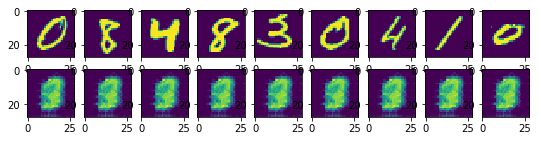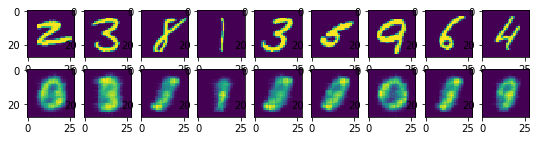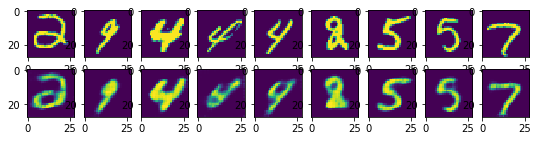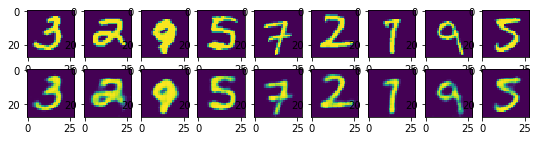At first, the reconstructed images look nothing like the originals. Rather, the reconstructions look more like the average of some training images. As training progresses, our reconstructions are clearer.

## Structure in the Embeddings¶

Since we are drastically reducing the dimensionality of the image, there has to be some kind of structure in the embedding space. That is, the network should be able to "save" space by mapping similar images to similar embeddings.

We will demonstrate the structure of the embedding space by hving some fun with our autoencoders. Let's begin with two images in our training set. For now, we'll choose images of the same digit.

In :
imgs = outputs[max_epochs-1].detach().numpy()
plt.subplot(1, 2, 1)
plt.imshow(imgs)
plt.subplot(1, 2, 2)
plt.imshow(imgs)

Out:
<matplotlib.image.AxesImage at 0x11da3c5c0>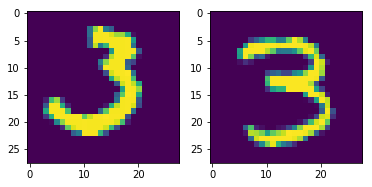We will then compute the low-dimensional embeddings of both images, by applying the encoder:

In :
x1 = outputs[max_epochs-1][0,:,:,:] # first image
x2 = outputs[max_epochs-1][8,:,:,:] # second image
x = torch.stack([x1,x2])     # stack them together so we only call encoder once
embedding = model.encoder(x)
e1 = embedding # embedding of first image
e2 = embedding # embedding of second image


Now we will do something interesting. Not only are we goign to run the decoder on those two embeddings e1 and e2, we are also going to interpolate between the two embeddings and decode those as well!

In :
embedding_values = []
for i in range(0, 10):
e = e1 * (i/10) + e2 * (10-i)/10
embedding_values.append(e)
embedding_values = torch.stack(embedding_values)

recons = model.decoder(embedding_values)


Let's plot the reconstructions of each interpolated values. The original images are shown below too:

In :
plt.figure(figsize=(10, 2))
for i, recon in enumerate(recons.detach().numpy()):
plt.subplot(2,10,i+1)
plt.imshow(recon)
plt.subplot(2,10,11)
plt.imshow(imgs)
plt.subplot(2,10,20)
plt.imshow(imgs)

Out:
<matplotlib.image.AxesImage at 0x11dd127f0>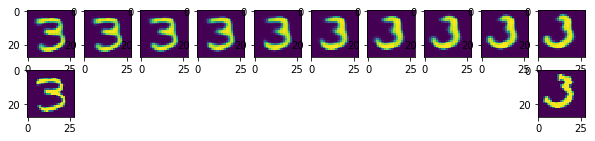Notice that there is a smooth transition between the two images! The middle images are likely new, in that there are no training images that are exactly like any of the generated images.

As promised, we can do the same thing with two images containing different digits. There should be a smooth transition between the two digits.

In :
def interpolate(index1, index2):
x1 = mnist_data[index1]
x2 = mnist_data[index2]
x = torch.stack([x1,x2])
embedding = model.encoder(x)
e1 = embedding # embedding of first image
e2 = embedding # embedding of second image

embedding_values = []
for i in range(0, 10):
e = e1 * (i/10) + e2 * (10-i)/10
embedding_values.append(e)
embedding_values = torch.stack(embedding_values)

recons = model.decoder(embedding_values)

plt.figure(figsize=(10, 2))
for i, recon in enumerate(recons.detach().numpy()):
plt.subplot(2,10,i+1)
plt.imshow(recon)
plt.subplot(2,10,11)
plt.imshow(x2)
plt.subplot(2,10,20)
plt.imshow(x1)

interpolate(0, 1)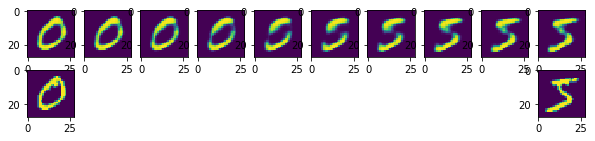In :
interpolate(1, 10)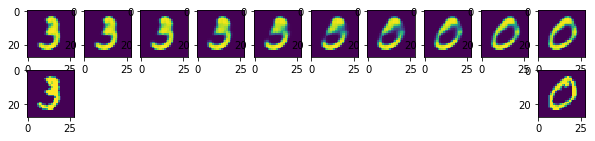In :
interpolate(4, 5)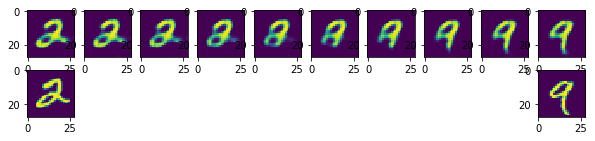In :
interpolate(20, 30)